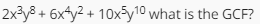# Factoring and Polynomials

This set of MCQs helps you brush up on important math topics and prepare you to dive into skill practice.

Start Quiz

What is the GCF? 12x+8

4

x

12

8

What is the GCF of 18k and 15k^3?

5k

3k^2

3k^3

3k

What is the value of k for which the given polynomial 2x^4−5x^3−2x^2−4x+k is divisible by x−3?

-2

3

2

12-x^3y^2

x^3y^2

2x^3y^2

2

Find factors of 2(2n^2+15)+29n.

(4n-5)(n-6)

(4n+5)(n-6)

(4n-5)(n+6)

(4n+5)(n+6)

Factorize:
(mx+ny)^2+(nx−my)^2

(m^2+n^2)(x^2-y^2)

(m^2+n^2)(x^2+y^2)

(m^2-n^2)(x^2+y^2)

(m^2-n^2)(x^2-y^2)

If f(x) and g(x) are two polynomials, which of the following may not be a polynomial?

f(x) - g(x)

f(x) / g(x)

f(x) * g(x)

f(x) *+g(x)

Factor
5ab^2c^11 + 2bc^5.

bc^5(5ab^2c^6 + 2b)

bc^5(5ab^2c^6 + b)

bc^5(5abc^6 - 2)

bc^5(5abc^6 + 2)

Quiz/Test Summary
Title: Factoring and Polynomials
Questions: 8
Contributed by: